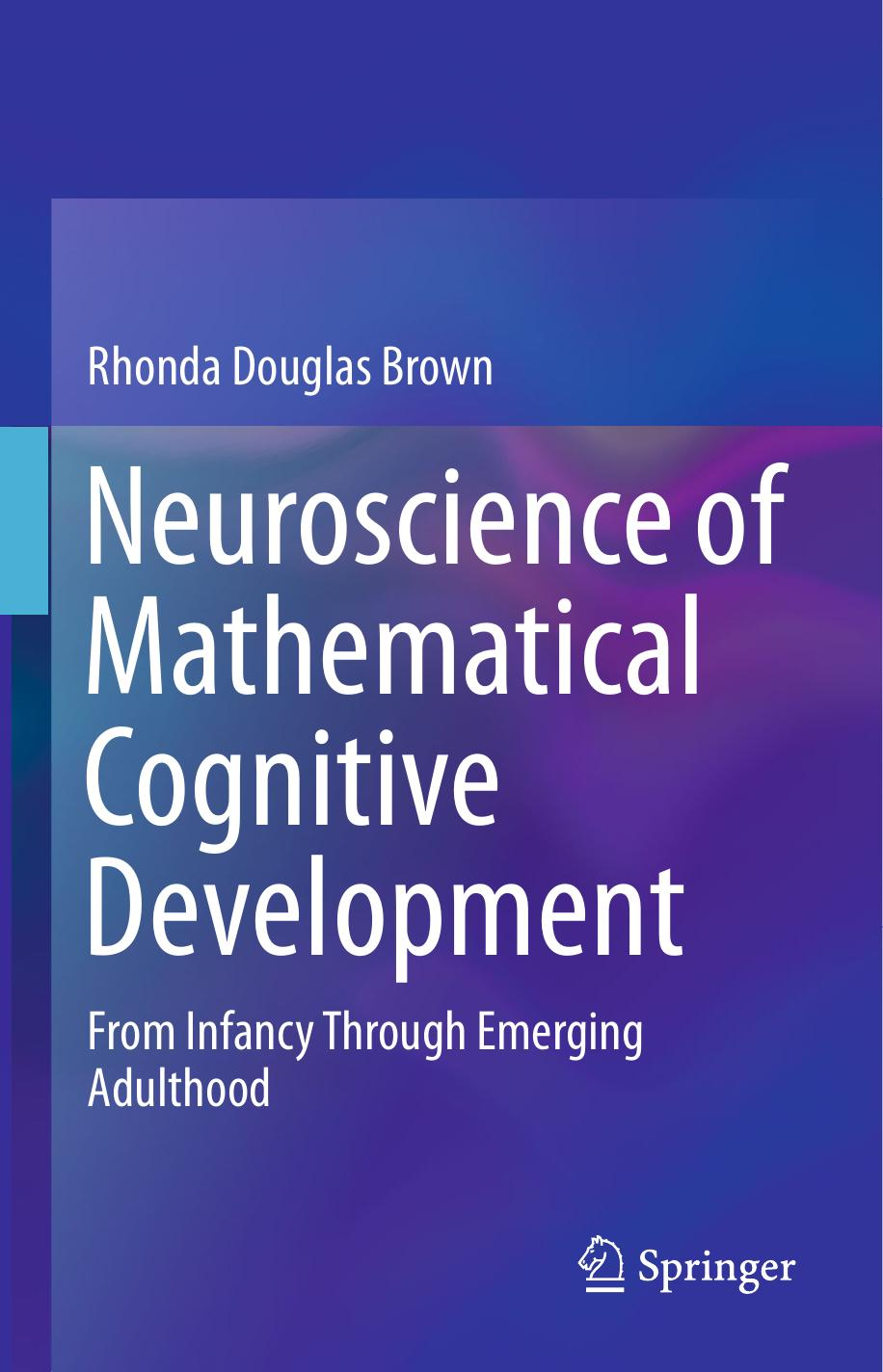﻿

# Neuroscience of Mathematical Cognitive Development by Rhonda Douglas BrownAuthor:Rhonda Douglas Brown
Language: eng
Format: epub
Publisher: Springer International Publishing, Cham

Approximate Calculation

Now, take a look at Fig. 4.9 and choose the closest answer to each problem.

Fig. 4.9Examples of problems used for three types of approximate calculation tasks: addition , subtraction , and multiplication

While undergoing fMRI , adolescents (N = 16) were presented with these types of problems and had 5 s to select the side of the screen displaying the closest answer. The problems involved 3-digit addition and subtraction , and 1-, 2-, or 3-digit multiplication with two possible answers, a reasonable estimate and one that was off by approximately 1 order of magnitude. To control for visual activation and response selection, we compared brain activation during mathematical problems to brain activation during a color matching task that gave adolescents 2.5 s to choose which of the two identical 2- or 3-digit numbers matched the color of the same number shown in the middle of the screen.

As shown in Fig. 4.10, activation in bilateral IPS and the left lingual gyrus during approximate calculation positively correlated with mathematics achievement. Activation in these areas plus the right inferior occipital gyrus positively correlated with calculation subtest scores. Significant negative approximate calculation task performance by subtest interactions for activation in right IPS were also found for math fluency and calculation.

Fig. 4.10Regions with significant (p < 0.01 FWE corrected) positive correlations between functional activation during approximate calculation and Woodcock-Johnson III percentile scores for math achievement and calculation . Regions with significant (p < 0.01 FWE corrected) negative approximate calculation task performance by math fluency and calculation percentile score interactions for functional activation. Images in radiologic orientation. Slice locations: Z −25 mm to Z = +70 mm